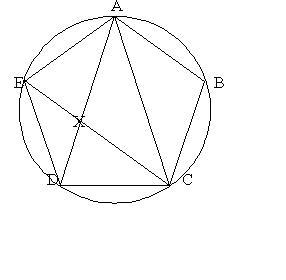#### You may also like### Kissing

Two perpendicular lines are tangential to two identical circles that touch. What is the largest circle that can be placed in between the two lines and the two circles and how would you construct it?### Gold Again

Without using a calculator, computer or tables find the exact values of cos36cos72 and also cos36 - cos72.### Golden Construction

Draw a square and an arc of a circle and construct the Golden rectangle. Find the value of the Golden Ratio.

# Pentabuild

##### Age 16 to 18Challenge LevelHere is some information about regular pentagons. $ABCDE$ is a regular pentagon. We first prove that triangles $AEX$ and $ADC$ are similar and that the ratio $AD/AE$ is equal to the golden ratio ${1+\sqrt 5\over 2}$.
As $ABCDE$ is a regular pentagon, triangles $AEX$ and $ADC$ have angles $36^o, 72^o, 72^o$ and so they are similar isosceles triangles. Label the side length of the pentagon $s$ so $AE=AX=CD=CX=s$ and the chord length $c$ so $AD=EC = c$ and $EX=c-s$. From the similar triangles $${c-s\over s}={s\over c}$$ so writing $AD/AE=c/s = x$ gives $$x - 1 = {1\over x}$$ which is the quadratic equation $x^2-x-1=0$ and hence, as it must be positive, the ratio $x=AD/AE$ is the golden ratio ${1+\sqrt 5\over 2}$.

Now we can find the exact values of $\cos 72^o$ and $\cos 36^o$.

Drawing a perpendicular from $E$ to $AD$ gives a right angled triangle from which $$\cos 36^o = {c\over 2s} ={\sqrt 5+1\over 4}.$$

Drawing a perpendicular from $A$ to $DC$ gives a right angled triangle from which $$\cos 72^o = {s\over 2c} ={1\over2\phi}={\sqrt 5-1\over 4}.$$

In explaining the construction of a regular pentagon coordinates are useful.

If the pentagon is inscribed in a unit circle with $A$ the point $(0,1)$ you can find the exact coordinates of $B$ and $C$ using the exact values of $\cos 72^o$ and $\cos 36^o$ which we have just found.

If the pentagon is inscribed in a unit circle with $A$ the point $(0,1)$ then $B=(\sin 72^o, \cos 72^o)$ and $C=(\sin 36^o, -\cos 36^o)$.

Hint: When you explain the construction focus on the $'y'$ coordinates of $B, C, D$ and $E$.